## Definition

The local radiation computes the radiation between surfaces. The surfaces can be emitters and receptors, and they are geometrically represented in the Software. So, they can exchange heat though the transparent environment (air or vacuum). This heat exchange, due to the bodies'temperature, is called thermal radiation .

It takes into account the geometrical configuration of each emitter and receptor elementary surface (distance, angle, area).

The following example shows some rays, emitted by the nodes 1 and 7:## Hypothesis

• The bodies participating in the radiation exchange are gray opaque bodies:
• Emissivity, absorption and reflection are taken into account
• The radiation is wave length independent
• All the bodies (radiating or not) are opaque: there is no transmission of the rays through the matter
• The rays are emitted and reflected in all the directionsNote: τ = 0

## View factors computation

To take into account the geometrical configuration of emitter and receptor elementary surfaces, the view factors are computed during solving process:

In 3D :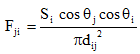In 2D :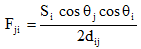## Thermal flux

After view factors computation, the thermal flux equation for the local radiation exchange is :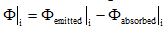With :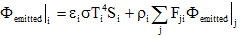and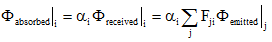ε is the emissivity coefficient,

σ is the StephanBoltzmann constant ( σ = 5,675 · 10-8 W/m2 /K4).

α is the absorption coefficient

ρ is the reflection coefficient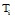is the node “i” temperature

## Access

The local radiation is available in all the thermal and thermal coupled applications, in 2D plane, 2D axisymmetric and 3D.

The local radiation information is defined, as for the radiation to infinite and the convection, on the linear regions in 2D (surface regions in 3D) of “region with surface of thermal exchange” type.

## Geometrical rebuilding & limitation

The geometrical rebuilding is managed in case of symmetries and periodicities.

But a limitation exists in axisymmetric: some rays of the non represented part can be ignored if obstacles exist.

## Mechanical sets

The view factors are computed at each solving step if mechanical sets exists in the project.

## Warnings

• For numerical reasons, the emitter and receptor surface elements must have a size smaller than the distance between the two surfaces.

For example, in case of studying the radiation at motor air gap, user has to pay attention on the air gap area mesh.

• The local radiation model works only if there is radiation exchange between surfaces (transfer in the two ways). A surface which does not receive thermal radiation flux, will not emit (if not, a body can loose energy until having negative temperature).